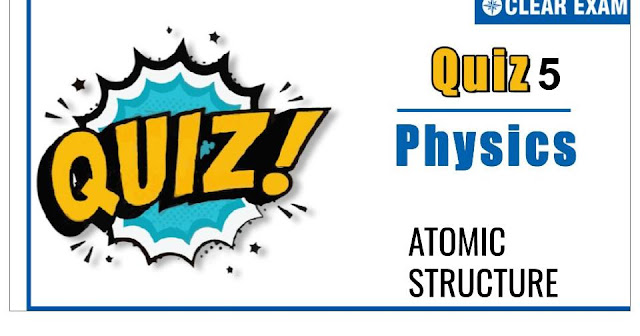## [LATEST]\$type=sticky\$show=home\$rm=0\$va=0\$count=4\$va=0

As per analysis for previous years, it has been observed that students preparing for NEET find Physics out of all the sections to be complex to handle and the majority of them are not able to comprehend the reason behind it. This problem arises especially because these aspirants appearing for the examination are more inclined to have a keen interest in Biology due to their medical background.

Furthermore, sections such as Physics are dominantly based on theories, laws, numerical in comparison to a section of Biology which is more of fact-based, life sciences, and includes substantial explanations. By using the table given below, you easily and directly access to the topics and respective links of MCQs. Moreover, to make learning smooth and efficient, all the questions come with their supportive solutions to make utilization of time even more productive. Students will be covered for all their studies as the topics are available from basics to even the most advanced.

Q1. The number of electrons and protons in an atoms of third alkaline earth metal is
•  e 20,p 20
•  e 18,p 20
•  e 18,p 18
•  e 19,p 20
Solution
The third alkaline metal is 〖_20^40〗Ca. It contains 20 protons and 20 electrons.

Q2.In ground state of chromium atom (Z=24) the total number of orbitals populated by one or more electrons is:
•  15
•  16
•  20
•  14
Solution
The configuration of _24 Cr is 1s^2,2s^2 2p^6,3s^2 3p^6 3d^5,4s^1 ∴ Total s-orbitals =4 Total p-orbitals =6 Total d-orbitals =5 and thus Total orbitals =4+6+5=15

Q3.  The energy per mole of photon of electromagnetic radiation of wavelength 4000 Å is:
•   3.0×10^(-12) erg
•  4.0×10^(-12) erg
•  5.0×10^(-12) erg
•  6.0×10^(-12) erg
Solution
E=(N∙hc)/λ

Q4. The number of electrons in an atom with atomic number 105 having (n+l)=8 are:
•  30
•  17
•  15
•  Unpredictable
Solution
The electronic configuration of element with at. no. 105 is: 1s^2,2s^2 2p^6,3s^2 3p^6 3d^10,4s^2 4p^6 4d^10 4f^14, 5s^2 5p^6 5d^10 5f^14,6s^2 6p^6 6d^3,7s^2 for 5f (n+l)=5+3=8 for 6d (n+l)=6+2=8

Q5.According to Bohr's theory, the angular momentum for an electron of 5th orbit is:
•  2.5h/π
•  5h/π
•  25h/π
•  6h/2π
Solution
Angular momentum=(n∙h)/2π=(5∙h)/2π=(2.5 h)/π .

Q6. The hydrogen spectrum from an incandescent source of hydrogen is:
•  A band spectrum in emission
•  A line spectrum in emission
•  A band spectrum in absorption
•  A line spectrum absorption
Solution
Elements show characteristics line spectrum which is finger print of atom.

Q7.The maximum number of 3d-electrons having spin quantum number s=+1/2 are:
•  10
•  14
•  5
•  None of these
Solution
3d-subshell has five orbitals. Each orbital can have one electron with spin +1/2.

Q8.Compared to the lightest atom the heaviest atom weighs:
•  200 times
•  238 times
•  92 times
•  16 times
Solution
Heaviest atom has mass no. 238,(i.e.,_92 U^238) and lighter one is 〖_1〗H^1.

Q9.Choose the arrangement which shows the increasing value of e/m for e,p,n and α-particles
•   n<α

•   e

•   n

•   p
Solution
Mass of electron =9.1×10^(-31) kg, Mass of proton =1.67×10^(-27) kg Mass of neutron =1.675×10^(-27) kg Mass of α-particle =6.67×10^(-27) kg So, increasing order of e/m for e,p,n and α-particle is e>p>α>n(∵ neutron has no charge)

Q10. The de Broglie wavelength associated with a material particle is:
•  Inversely proportional to momentum
•  Inversely proportional to its energy
•  Directly proportional to momentum
•  Directly proportional to its energy
Solution
de Broglie equation is λ=h/mu#### Written by: AUTHORNAME

AUTHORDESCRIPTION## Want to know more

Please fill in the details below:

## Latest NEET Articles\$type=three\$c=3\$author=hide\$comment=hide\$rm=hide\$date=hide\$snippet=hide

Name

ltr
item
BEST NEET COACHING CENTER | BEST IIT JEE COACHING INSTITUTE | BEST NEET & IIT JEE COACHING: ATOMIC STRUCTURE QUIZ-5
ATOMIC STRUCTURE QUIZ-5
https://1.bp.blogspot.com/-bNlSW_y4Y58/X7AzphZrs7I/AAAAAAAANxg/g34om1cMhHEVbIYDEXQ8DgbYQ4cKUeLPQCLcBGAsYHQ/w640-h336/Quiz%2BImage%2B20%2B%25284%2529.jpg
https://1.bp.blogspot.com/-bNlSW_y4Y58/X7AzphZrs7I/AAAAAAAANxg/g34om1cMhHEVbIYDEXQ8DgbYQ4cKUeLPQCLcBGAsYHQ/s72-w640-c-h336/Quiz%2BImage%2B20%2B%25284%2529.jpg
BEST NEET COACHING CENTER | BEST IIT JEE COACHING INSTITUTE | BEST NEET & IIT JEE COACHING
https://www.cleariitmedical.com/2020/11/atomic%20structure%20quiz%205.html
https://www.cleariitmedical.com/
https://www.cleariitmedical.com/
https://www.cleariitmedical.com/2020/11/atomic%20structure%20quiz%205.html
true
7783647550433378923
UTF-8

STAY CONNECTED